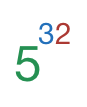# Just Count: 3,6,9, and so on.

Algebra Level 1How many digits are there in the number: $23,283,064,365,386,962,890,625$?

Details and Assumptions

• You may use the fact the number factors to $5^{32}$

• You may use the approximation: $\log_{10} 5 \approx 0.69897$

Try this

×